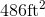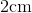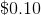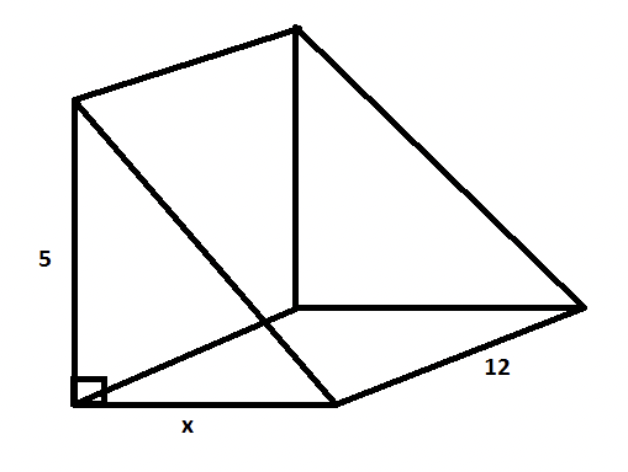# ACT Mathematics: Coordinate Geometry and Solid Geometry Quiz

This is an MCQ-based quiz on the topic of ACT Mathematics: Coordinate Geometry and Solid Geometry Quiz.

This set of MCQs helps you brush up on the important mathematics topic and prepare you to dive into skill practice and expand your knowledge to tackle the question and answer it carefully.

Start Quiz

A pyramid is placed inside a cube so that they share a base and height. If the surface area of the cube is, what is the volume of the pyramid, in square feet?

216

225

729

243

Troy's company manufactures dice that are shaped like cubes and have side lengths of. If the plastic needed to make the dice costs\$0.10 per cubic centimeter, how much does it cost Troy to make one die?

0.4

0.6

0.8

1.6

The height of a box is twice its width and half its length. If the volume of the box is 64 yd^3, what is the length of the box?

4

2

6

8

The volume of the right triangular prism is 120. Find the value of x.3

4

2

6

Matt baked a rectangular cake for his mom's birthday. The cake was 15 inches long, 12 inches wide, and 4 inches high. If he cuts the cake into pieces that are 3 inches long, 2 inches wide, and 4 inches high, how many pieces of cake can he cut?

30

28

40

24

If we have a regular (the triangles are equilateral) triangular prism of volume 32in^3 and the side length of the triangle on either face is 3in, what is the length of the prism? Write your answer in terms of a decimal rounded to the nearest hundredth.

12.32

3.56

10.67

2.56

Find the equation of a tangent line to

y=x^2

for the point

(0,0) ?

y = 0

y = 2

y = 1

y = 2x

What is the equation of a tangent line to

y=x^2+4x+2

at point (1,7) ?

y=6x+1

y=6x+9

y=6x-1

y=6x-9

Give the equation, in slope-intercept form, of the line tangent to the circle of the equation

x^2−6x+y^2−91=0

at the point (−3,8).

y = 3/4x + 41/4

y = 3/4x + 41/6

y = -3/4x + 41/4

y = 3/4x - 41/4

Circle A is centered about the origin and has a radius of 5. What is the equation of the line that is tangent to Circle A at the point (–3,4)?

3x – 4y = –25

3x – 4y = 25

3x + 4y = –25

-3x + 4y = –29

Quiz/Test Summary
Title: ACT Mathematics: Coordinate Geometry and Solid Geometry Quiz
Questions: 10
Contributed by: